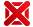Computability - An Introduction To Recursive Function Theory - 9780521294652

Categorias: Idiomas | Inglês

Computability - An Introduction To Recursive Function Theory Cutland, Nigel J. Cambridge Usa
Avaliações

Por: R\$ 448,50

em até 4x sem juros no cartãoProduto indisponívelEste produto está temporariamente indisponível.
Nós avisaremos quando ele chegar.

Sinopse

What can computers do in principle? What are their inherent theoretical limitations? These are questions to which computer scientists must address themselves. The theoretical framework which enables such questions to be answered has been developed over the last fifty years from the idea of a computable function: intuitively a function whose values can be calculated in an effective or automatic way. This book is an introduction to computability theory (or recursion theory as it is traditionally known to mathematicians). Dr Cutland begins with a mathematical characterisation of computable functions using a simple idealised computer (a register machine); after some comparison with other characterisations, he develops the mathematical theory, including a full discussion of non-computability and undecidability, and the theory of recursive and recursively enumerable sets. The later chapters provide an introduction to more advanced topics such as Gildel´s incompleteness theorem, degrees of unsolvability, the Recursion theorems and the theory of complexity of computation. Computability is thus a branch of mathematics which is of relevance also to computer scientists and philosophers. Mathematics students with no prior knowledge of the subject and computer science students who wish to supplement their practical expertise with some theoretical background will find this book of use and interest.

Detalhes do produto

 Peso: 0,362 kg Número de páginas: 264 Ano de edição: 1980 ISBN 10: 0521294657 ISBN 13: 9780521294652 Altura: 22 Largura: 15 Comprimento: 1 Idioma : Inglês Tipo de produto : Livro Assuntos : Ciências Exatas Assuntos : Matemática